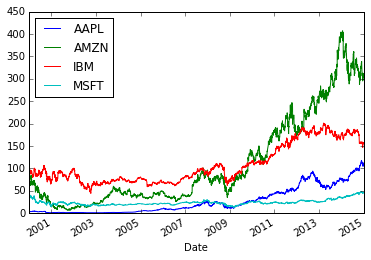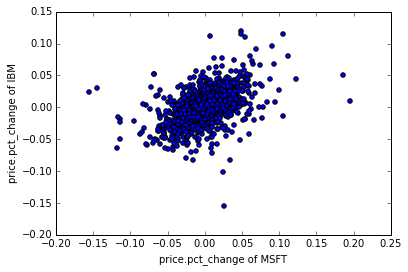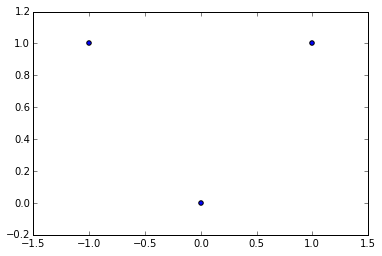## Correlations¶

### Measuring associations between random variables¶

In :
%matplotlib inline
import numpy as np

In :
cov = np.array([[2.0, 0.8], [0.8, 0.6]])
mean = np.array([2., 1.])

rd = np.random.multivariate_normal(mean, cov, 50)
x = rd[:,0]


### Mean¶

$$\bar x = \frac{\sum_{i=1}^{m} x_i}{m}$$
• $x_i$: i-th data point
• $m$: number of data points
In :
x.mean()

Out:
2.1553095098460919

### Standard deviation¶

Standard deviation: "Average distance of the mean to a data point"

$$\sigma_x = \sqrt \frac{\sum_{i=1}^{m} (\bar x - x_i)^2}{m-1}$$

Estimated mean "sees" a smaller spread (hand waving argument for (m-1) instead of m in denominator.

• mean is known rather than estimated
• samples are known to come from a Gaussian (gives max-linkelihood estimator)
In :
# denominator m
print np.sqrt(((x-x.mean())**2).mean())
print x.std()

# denominator m-1
print np.sqrt( ((x-x.mean())**2).sum()/(len(x)-1.) )
print x.std(ddof=1)

1.49002460382
1.49002460382
1.50515214499
1.50515214499


### Variance¶

$$\sigma_x^2 = \frac{\sum_{i=1}^{m} (\bar x - x_i)^2}{m-1} = \frac{\sum_{i=1}^{m} (\bar x - x_i)(\bar x - x_i)}{m-1}$$
In :
x.var()

Out:
1.8528546471199692
In :
x=rd


### Covariance¶

$$cov(x,y) = \frac{\sum_{i=1}^{m} (\bar x - x_i)(\bar y - y_i)}{m-1}$$

### Covariance matrix¶

$$C = \left(\begin{array}{ccc} cov(x_1,x_1) & cov(x_1,x_2) & cov(x_1,x_3) \\ cov(x_2,x_1) & cov(x_2,x_2) & cov(x_2,x_3) \\ cov(x_3,x_1) & cov(x_3,x_2) & cov(x_3,x_3) \end{array}\right)$$

Note: $C = C^T$ (cov is symetric)

In :
np.cov(x.T)

Out:
array([[ 2.01014683,  0.87391461],
[ 0.87391461,  0.66372703]])

### (Pearson) Correlation¶

$$\rho_{xy} = \frac {cov(x, y)}{\sigma_x \sigma_y}$$
In :
np.corrcoef(x.T)

Out:
array([[ 1.        ,  0.60671013],
[ 0.60671013,  1.        ]])

$-1 \leq \rho \leq 1$

$\rho > 0$ : $x$ and $y$ are correlated
$\rho < 0$ : $x$ and $y$ are anti-correlated
$\rho \approx 0$ : (linear) uncorrelated

For what level of measurement is the Pearson Correlation adequat?

In :
#example from book "python for data analysis"
import pandas.io.data as web
import pandas as pd

all_data = {}
for ticker in ['IBM', 'MSFT', 'AAPL', 'AMZN']: # google doesn't work?? 'GOOG']:
all_data[ticker] = web.get_data_yahoo(ticker, '1/1/2000', '1/5/2015')

price = pd.DataFrame({tic: data['Adj Close'] for tic, data in all_data.iteritems()})

volume = pd.DataFrame({tic: data['Volume'] for tic, data in all_data.iteritems()})

price.plot()

Out:
<matplotlib.axes._subplots.AxesSubplot at 0x11be78690>In :
# Percent change over given number of periods.
returns = price.pct_change()
returns.tail(9)

Out:
AAPL AMZN IBM MSFT
Date
2014-12-22 0.010378 0.022141 0.018485 0.006714
2014-12-23 -0.003542 -0.000816 0.004955 0.009796
2014-12-24 -0.004709 -0.010644 -0.002589 -0.006398
2014-12-26 0.017677 0.019998 0.003213 -0.005401
2014-12-29 -0.000702 0.009544 -0.011273 -0.008981
2014-12-30 -0.012203 -0.005576 -0.002866 -0.009062
2014-12-31 -0.019019 0.000161 0.002437 -0.012122
2015-01-02 -0.009513 -0.005897 0.010097 0.006674
2015-01-05 -0.028172 -0.020517 -0.015735 -0.009196
In :
import matplotlib.pyplot as plt

plt.scatter(returns.MSFT, returns.IBM)
plt.xlabel('price.pct_change of MSFT')
plt.ylabel('price.pct_change of IBM')

Out:
<matplotlib.text.Text at 0x11be82a90>In :
#correlations coeffient
returns.MSFT.corr(returns.IBM)

Out:
0.49627848345294678
In :
returns.corr()

Out:
AAPL AMZN IBM MSFT
AAPL 1.000000 0.327467 0.404268 0.412829
AMZN 0.327467 1.000000 0.320703 0.382260
IBM 0.404268 0.320703 1.000000 0.496278
MSFT 0.412829 0.382260 0.496278 1.000000
In :
returns.cov()

Out:
AAPL AMZN IBM MSFT
AAPL 0.000778 0.000333 0.000192 0.000233
AMZN 0.000333 0.001327 0.000199 0.000281
IBM 0.000192 0.000199 0.000290 0.000171
MSFT 0.000233 0.000281 0.000171 0.000409
In :
returns.corrwith(returns.IBM)

Out:
AAPL    0.404268
AMZN    0.320703
IBM     1.000000
MSFT    0.496278
dtype: float64
In :
returns.corrwith(volume)

Out:
AAPL   -0.061123
AMZN    0.137429
IBM    -0.037555
MSFT   -0.032484
dtype: float64

Pearsons correlation coefficient is only sensitiv for linear correlations:

In :
from IPython.display import SVG, display


Independent implies zero correlation, but not vice versa:

$$x \bot y \Leftrightarrow P(x,y) = P(x) P(y) \Rightarrow cov(x,y) = 0$$

but $$x \bot y \nLeftarrow cov(x,y) = 0$$

Analytic example:

In :
x = np.array([-1,0,1])
y = x**2 # deterministic
d = np.concatenate((x[:,None],y[:,None]), axis=1)
print np.cov(d.T)
plt.scatter(d[:,0], d[:,1])

[[ 1.          0.        ]
[ 0.          0.33333333]]

Out:
<matplotlib.collections.PathCollection at 0x11a567810>### Rank correlation coefficients¶

• Spearmen correlation coefficient
• Kendall $\tau$

### Distance covariance and distance correlations¶

(non-linear correlation coefficent)

"You take all pairwise distances between sample values of one variable, and do the same for the second variable. Then center the resulting distance matrices (so each has column and row means equal to zero) and average the entries of the matrix which holds componentwise products of the two centered distance matrices. That’s the squared distance covariance between the two variables. The population quantity equals zero if and only if the variables are independent, whatever be the underlying distributions and whatever be the dimen- sion of the two variables. " INTRODUCING THE DISCUSSION PAPER BY SZÉKELY AND RIZZO, MICHAEL A. NEWTON